Courses

# Shortest Paths Computer Science Engineering (CSE) Notes | EduRev

## Computer Science Engineering (CSE) : Shortest Paths Computer Science Engineering (CSE) Notes | EduRev

The document Shortest Paths Computer Science Engineering (CSE) Notes | EduRev is a part of the Computer Science Engineering (CSE) Course GATE Computer Science Engineering(CSE) 2022 Mock Test Series.
All you need of Computer Science Engineering (CSE) at this link: Computer Science Engineering (CSE)

## Bellman–Ford Algorithm)

Given a graph and a source vertex src in graph, find shortest paths from src to all vertices in the given graph. The graph may contain negative weight edges.
We have discussed Dijkstra`s algorithm for this problem. Dijksra’s algorithm is a Greedy algorithm and time complexity is O(VLogV) (with the use of Fibonacci heap). Dijkstra doesn’t work for Graphs with negative weight edges, Bellman-Ford works for such graphs. Bellman-Ford is also simpler than Dijkstra and suites well for distributed systems. But time complexity of Bellman-Ford is O(VE), which is more than Dijkstra.

Algorithm

Following are the detailed steps.

Input: Graph and a source vertex src
Output: Shortest distance to all vertices from src. If there is a negative weight cycle, then shortest distances are not calculated, negative weight cycle is reported.

1) This step initializes distances from source to all vertices as infinite and distance to source itself as 0. Create an array dist[] of size |V| with all values as infinite except dist[src] where src is source vertex.

2) This step calculates shortest distances. Do following |V|-1 times where |V| is the number of vertices in given graph.
…..a) Do following for each edge u-v
………………If dist[v] > dist[u] + weight of edge uv, then update dist[v]
………………….dist[v] = dist[u] + weight of edge uv

3) This step reports if there is a negative weight cycle in graph. Do following for each edge u-v
……If dist[v] > dist[u] + weight of edge uv, then “Graph contains negative weight cycle”
The idea of step 3 is, step 2 guarantees shortest distances if graph doesn’t contain negative weight cycle. If we iterate through all edges one more time and get a shorter path for any vertex, then there is a negative weight cycle

How does this work?

Like other Dynamic Programming Problems, the algorithm calculate shortest paths in bottom-up manner. It first calculates the shortest distances for the shortest paths which have at-most one edge in the path. Then, it calculates shortest paths with at-nost 2 edges, and so on. After the ith iteration of outer loop, the shortest paths with at most i edges are calculated. There can be maximum |V| – 1 edges in any simple path, that is why the outer loop runs |v| – 1 times. The idea is, assuming that there is no negative weight cycle, if we have calculated shortest paths with at most i edges, then an iteration over all edges guarantees to give shortest path with at-most (i+1) edges.

Example
Let us understand the algorithm with following example graph.

Let the given source vertex be 0. Initialize all distances as infinite, except the distance to source itself. Total number of vertices in the graph is 5, so all edges must be processed 4 times.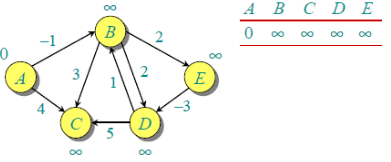Let all edges are processed in following order: (B,E), (D,B), (B,D), (A,B), (A,C), (D,C), (B,C), (E,D). We get following distances when all edges are processed first time. The first row in shows initial distances. The second row shows distances when edges (B,E), (D,B), (B,D) and (A,B) are processed. The third row shows distances when (A,C) is processed. The fourth row shows when (D,C), (B,C) and (E,D) are processed.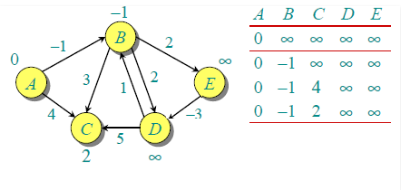The first iteration guarantees to give all shortest paths which are at most 1 edge long. We get following distances when all edges are processed second time (The last row shows final values).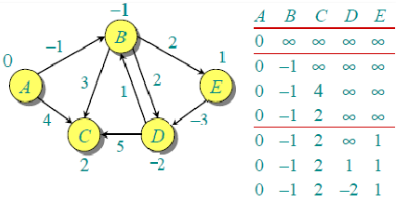The second iteration guarantees to give all shortest paths which are at most 2 edges long. The algorithm processes all edges 2 more times. The distances are minimized after the second iteration, so third and fourth iterations don’t update the distances.

Implementation:

// A C / C++ program for Bellman-Ford's single source
// shortest path algorithm.

#include <stdio.h>
#include <stdlib.h>
#include <string.h>
#include <limits.h>

// a structure to represent a weighted edge in graph
struct Edge
{
int src, dest, weight;
};

// a structure to represent a connected, directed and
// weighted graph
struct Graph
{
// V-> Number of vertices, E-> Number of edges
int V, E;

// graph is represented as an array of edges.
struct Edge* edge;
};

// Creates a graph with V vertices and E edges
struct Graph* createGraph(int V, int E)
{
struct Graph* graph =
(struct Graph*) malloc( sizeof(struct Graph) );
graph->V = V;
graph->E = E;

graph->edge =
(struct Edge*) malloc( graph->E * sizeof( struct Edge ) );

return graph;
}

// A utility function used to print the solution
void printArr(int dist[], int n)
{
printf("Vertex   Distance from Source ");
for (int i = 0; i < n; ++i)
printf("%d %d ", i, dist[i]);
}

// The main function that finds shortest distances from src to
// all other vertices using Bellman-Ford algorithm.  The function
// also detects negative weight cycle
void BellmanFord(struct Graph* graph, int src)
{
int V = graph->V;
int E = graph->E;
int dist[V];

// Step 1: Initialize distances from src to all other vertices
// as INFINITE
for (int i = 0; i < V; i++)
dist[i]   = INT_MAX;
dist[src] = 0;

// Step 2: Relax all edges |V| - 1 times. A simple shortest
// path from src to any other vertex can have at-most |V| - 1
// edges
for (int i = 1; i <= V-1; i++)
{
for (int j = 0; j < E; j++)
{
int u = graph->edge[j].src;
int v = graph->edge[j].dest;
int weight = graph->edge[j].weight;
if (dist[u] != INT_MAX && dist[u] + weight < dist[v])
dist[v] = dist[u] + weight;
}
}

// Step 3: check for negative-weight cycles.  The above step
// guarantees shortest distances if graph doesn't contain
// negative weight cycle.  If we get a shorter path, then there
// is a cycle.
for (int i = 0; i < E; i++)
{
int u = graph->edge[i].src;
int v = graph->edge[i].dest;
int weight = graph->edge[i].weight;
if (dist[u] != INT_MAX && dist[u] + weight < dist[v])
printf("Graph contains negative weight cycle");
}

printArr(dist, V);

return;
}

// Driver program to test above functions
int main()
{
/* Let us create the graph given in above example */
int V = 5;  // Number of vertices in graph
int E = 8;  // Number of edges in graph
struct Graph* graph = createGraph(V, E);

// add edge 0-1 (or A-B in above figure)
graph->edge.src = 0;
graph->edge.dest = 1;
graph->edge.weight = -1;

// add edge 0-2 (or A-C in above figure)
graph->edge.src = 0;
graph->edge.dest = 2;
graph->edge.weight = 4;

// add edge 1-2 (or B-C in above figure)
graph->edge.src = 1;
graph->edge.dest = 2;
graph->edge.weight = 3;

// add edge 1-3 (or B-D in above figure)
graph->edge.src = 1;
graph->edge.dest = 3;
graph->edge.weight = 2;

// add edge 1-4 (or A-E in above figure)
graph->edge.src = 1;
graph->edge.dest = 4;
graph->edge.weight = 2;

// add edge 3-2 (or D-C in above figure)
graph->edge.src = 3;
graph->edge.dest = 2;
graph->edge.weight = 5;

// add edge 3-1 (or D-B in above figure)
graph->edge.src = 3;
graph->edge.dest = 1;
graph->edge.weight = 1;

// add edge 4-3 (or E-D in above figure)
graph->edge.src = 4;
graph->edge.dest = 3;
graph->edge.weight = -3;

BellmanFord(graph, 0);

return 0;
}

Output:
Vertex   Distance from Source
0                0
1                -1
2                2
3                -2
4                1

Notes
1) Negative weights are found in various applications of graphs. For example, instead of paying cost for a path, we may get some advantage if we follow the path.

2) Bellman-Ford works better (better than Dijksra’s) for distributed systems. Unlike Dijksra’s where we need to find minimum value of all vertices, in Bellman-Ford, edges are considered one by one.

Exercise
1) The standard Bellman-Ford algorithm reports shortest path only if there is no negative weight cycles. Modify it so that it reports minimum distances even if there is a negative weight cycle.

2) Can we use Dijksra’s algorithm for shortest paths for graphs with negative weights – one idea can be, calculate the minimum weight value, add a positive value (equal to absolute value of minimum weight value) to all weights and run the Dijksra’s algorithm for the modified graph. Will this algorithm work?

## Dijkstra’s shortest path algorithm

Given a graph and a source vertex in graph, find shortest paths from source to all vertices in the given graph.

Dijkstra’s algorithm is very similar to Prim`s algorithm for minimum spanning tree. Like Prim’s MST, we generate a SPT (shortest path tree) with given source as root. We maintain two sets, one set contains vertices included in shortest path tree, other set includes vertices not yet included in shortest path tree. At every step of the algorithm, we find a vertex which is in the other set (set of not yet included) and has minimum distance from source.

Below are the detailed steps used in Dijkstra’s algorithm to find the shortest path from a single source vertex to all other vertices in the given graph.
Algorithm
1) Create a set sptSet (shortest path tree set) that keeps track of vertices included in shortest path tree, i.e., whose minimum distance from source is calculated and finalized. Initially, this set is empty.
2) Assign a distance value to all vertices in the input graph. Initialize all distance values as INFINITE. Assign distance value as 0 for the source vertex so that it is picked first.
3) While sptSet doesn’t include all vertices
….a) Pick a vertex u which is not there in sptSetand has minimum distance value.
….b) Include u to sptSet.
….c) Update distance value of all adjacent vertices of u. To update the distance values, iterate through all adjacent vertices. For every adjacent vertex v, if sum of distance value of u (from source) and weight of edge u-v, is less than the distance value of v, then update the distance value of v.

Let us understand with the following example: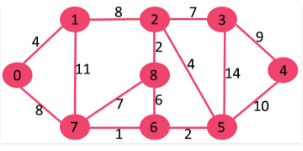The set sptSetis initially empty and distances assigned to vertices are {0, INF, INF, INF, INF, INF, INF, INF} where INF indicates infinite. Now pick the vertex with minimum distance value. The vertex 0 is picked, include it in sptSet. So sptSet becomes {0}. After including 0 to sptSet, update distance values of its adjacent vertices. Adjacent vertices of 0 are 1 and 7. The distance values of 1 and 7 are updated as 4 and 8. Following subgraph shows vertices and their distance values, only the vertices with finite distance values are shown. The vertices included in SPT are shown in green color.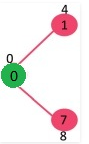Pick the vertex with minimum distance value and not already included in SPT (not in sptSET). The vertex 1 is picked and added to sptSet. So sptSet now becomes {0, 1}. Update the distance values of adjacent vertices of 1. The distance value of vertex 2 becomes 12.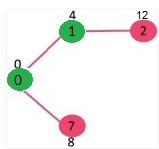Pick the vertex with minimum distance value and not already included in SPT (not in sptSET). Vertex 7 is picked. So sptSet now becomes {0, 1, 7}. Update the distance values of adjacent vertices of 7. The distance value of vertex 6 and 8 becomes finite (15 and 9 respectively).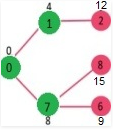Pick the vertex with minimum distance value and not already included in SPT (not in sptSET). Vertex 6 is picked. So sptSet now becomes {0, 1, 7, 6}. Update the distance values of adjacent vertices of 6. The distance value of vertex 5 and 8 are updated.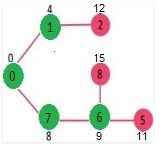We repeat the above steps until sptSet doesn’t include all vertices of given graph. Finally, we get the following Shortest Path Tree (SPT).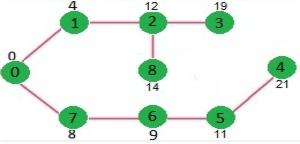We use a boolean array sptSet[] to represent the set of vertices included in SPT. If a value sptSet[v] is true, then vertex v is included in SPT, otherwise not. Array dist[] is used to store shortest distance values of all vertices.

// A C / C++ program for Dijkstra's single source shortest path algorithm.
// The program is for adjacency matrix representation of the graph

#include <stdio.h>
#include <limits.h>

// Number of vertices in the graph
#define V 9

// A utility function to find the vertex with minimum distance value, from
// the set of vertices not yet included in shortest path tree
int minDistance(int dist[], bool sptSet[])
{
// Initialize min value
int min = INT_MAX, min_index;

for (int v = 0; v < V; v++)
if (sptSet[v] == false && dist[v] <= min)
min = dist[v], min_index = v;

return min_index;
}

// A utility function to print the constructed distance array
int printSolution(int dist[], int n)
{
printf("Vertex   Distance from Source ");
for (int i = 0; i < V; i++)
printf("%d %d ", i, dist[i]);
}

// Funtion that implements Dijkstra's single source shortest path algorithm
// for a graph represented using adjacency matrix representation
void dijkstra(int graph[V][V], int src)
{
int dist[V];     // The output array.  dist[i] will hold the shortest
// distance from src to i

bool sptSet[V]; // sptSet[i] will true if vertex i is included in shortest
// path tree or shortest distance from src to i is finalized

// Initialize all distances as INFINITE and stpSet[] as false
for (int i = 0; i < V; i++)
dist[i] = INT_MAX, sptSet[i] = false;

// Distance of source vertex from itself is always 0
dist[src] = 0;

// Find shortest path for all vertices
for (int count = 0; count < V-1; count++)
{
// Pick the minimum distance vertex from the set of vertices not
// yet processed. u is always equal to src in first iteration.
int u = minDistance(dist, sptSet);

// Mark the picked vertex as processed
sptSet[u] = true;

// Update dist value of the adjacent vertices of the picked vertex.
for (int v = 0; v < V; v++)

// Update dist[v] only if is not in sptSet, there is an edge from
// u to v, and total weight of path from src to  v through u is
// smaller than current value of dist[v]
if (!sptSet[v] && graph[u][v] && dist[u] != INT_MAX
&& dist[u]+graph[u][v] < dist[v])
dist[v] = dist[u] + graph[u][v];
}

// print the constructed distance array
printSolution(dist, V);
}

// driver program to test above function
int main()
{
/* Let us create the example graph discussed above */
int graph[V][V] = {{0, 4, 0, 0, 0, 0, 0, 8, 0},
{4, 0, 8, 0, 0, 0, 0, 11, 0},
{0, 8, 0, 7, 0, 4, 0, 0, 2},
{0, 0, 7, 0, 9, 14, 0, 0, 0},
{0, 0, 0, 9, 0, 10, 0, 0, 0},
{0, 0, 4, 14, 10, 0, 2, 0, 0},
{0, 0, 0, 0, 0, 2, 0, 1, 6},
{8, 11, 0, 0, 0, 0, 1, 0, 7},
{0, 0, 2, 0, 0, 0, 6, 7, 0}
};

dijkstra(graph, 0);

return 0;
}

Output:
Vertex   Distance from Source
0                0
1                4
2                12
3                19
4                21
5                11
6                9
7                8
8                14

Notes:
1) The code calculates shortest distance, but doesn’t calculate the path information. We can create a parent array, update the parent array when distance is updated (like prim`s implementation) and use it show the shortest path from source to different vertices.

2) The code is for undirected graph, same dijkstra function can be used for directed graphs also.

3) The code finds shortest distances from source to all vertices. If we are interested only in shortest distance from source to a single target, we can break the for loop when the picked minimum distance vertex is equal to target (Step 3.a of algorithm).

4) Time Complexity of the implementation is O(V^2). If the input graph is represented using adjacency list, it can be reduced to O(E log V) with the help of binary heap.

5) Dijkstra’s algorithm doesn’t work for graphs with negative weight edges. For graphs with negative weight edges, Bellman-Ford algorithm can be used, we will soon be discussing it as a separate post.

Offer running on EduRev: Apply code STAYHOME200 to get INR 200 off on our premium plan EduRev Infinity!

## GATE Computer Science Engineering(CSE) 2022 Mock Test Series

131 docs|159 tests

,

,

,

,

,

,

,

,

,

,

,

,

,

,

,

,

,

,

,

,

,

;